# Design and Analyze Tapered-Slot SIW Filtenna

This example shows how to create the substrate-integrated-waveguide-based (SIW-based) antipodal filtenna described in  with a modified feeding network. A filtenna is a planar antenna with a built-in filter, which can be used to provide frequency agility for communicating at different frequencies without causing any interference to the adjacent bands.You can also use a filtenna to avoid undesired frequencies when you use it as a receiver.

The SIW filtenna design in this example has an operating range of 23.8 GHz to 40 GHz, maximum gain of 10.25 dB, and a maximum out-of-band suppression of 20.55 dB.

This figure shows the top view of the filtenna.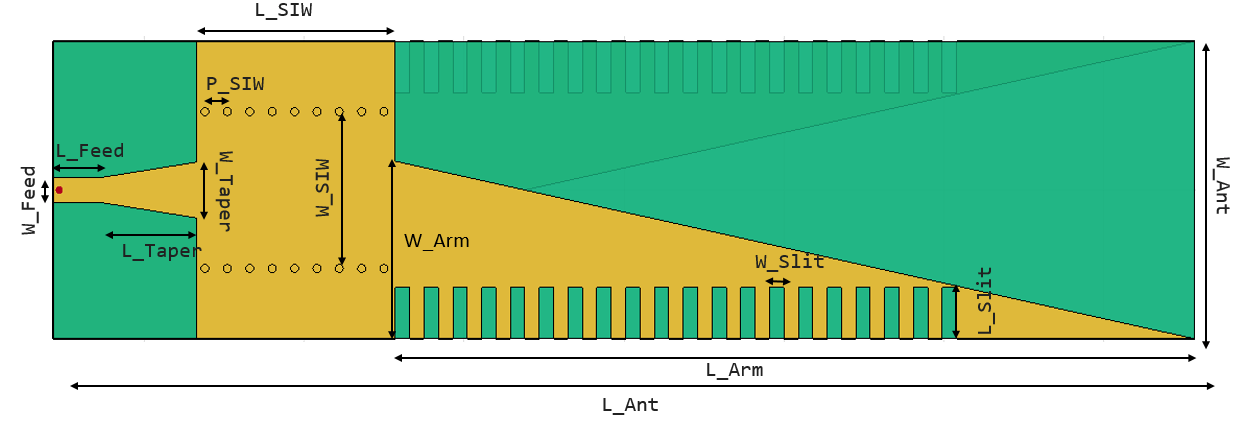This figure shows the bottom view of the filtenna.

.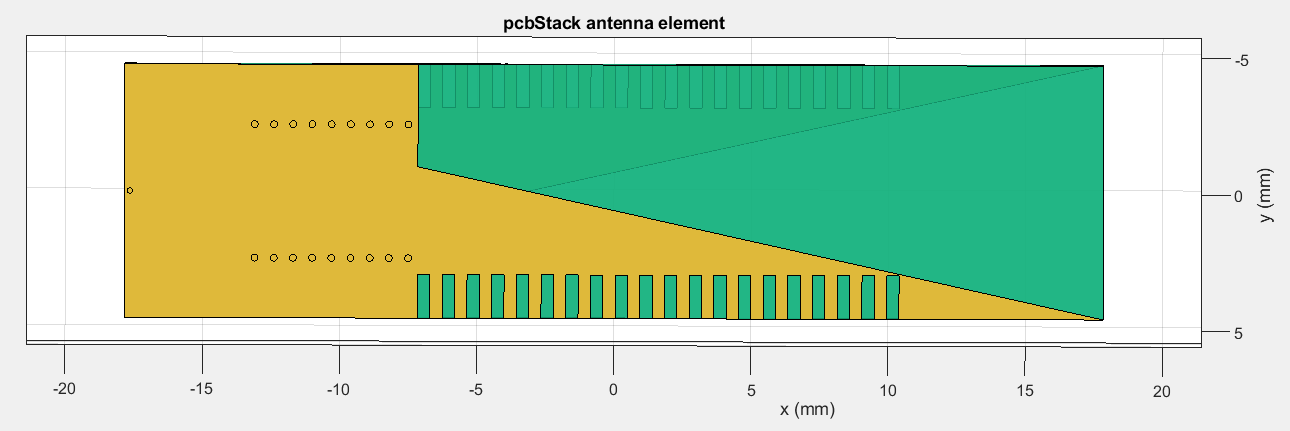### Create SIW Filtenna

Define the SIW patch, radiator, taper, feedline, ground plane, and slit dimensions as well as slit and via locations.

```l_siw = 6.2e-3; % SIW patch length l_arm = 25e-3; % Radiator length l_taper = 3e-3; % Taper length l_feed = 1.5e-3; % Feedline length w_feed = 0.7826e-3; % Feedline width w_taper = 1.745e-3; % Taper width w_ant = 9.3e-3; % Antenna width w_siw = 4.9e-3; % SIW patch width w_arm = 5.55e-3; % Radiator and ground plane width l_gnd = l_siw + l_taper + l_feed; % Ground plane length in the botto layer w_gnd = w_ant; % Ground plane width l_ant = l_feed + l_taper + l_siw + l_arm; % Total length of the antenna viaX = -l_ant/2 + l_feed + l_taper + 0.25e-3; % Via start point location on x-axis viaY = w_siw/2; % Via start point location on y-axis p_siw = 0.7e-3; % Via pitch l_slit = 0.45e-3; % Side slit length w_slit = 1.6e-3; % Side slit width slitX = -l_ant/2 + l_feed + l_taper + l_siw + l_slit/2; % X-coordinate of slit position slitY = -w_ant/2 + w_slit/2; % Y-coordinate of slit position```

#### Create Tapered Microstrip Line

Use the specified dimesions to create a microstrip transmission line. Use a tapered section to match the impedance with a 50 Ω microstrip line in which the quasi-TEM and TE10 modes are dominant. Create a microstrip line up to the SIW transition trace. Visualize the transition using the `show` method.

```feedline = antenna.Rectangle(Length=l_feed,Width=w_feed,Center=[-l_ant/2+l_feed/2 0]); taper = antenna.Polygon; taper.Name = "taper"; taper.Vertices = [-l_ant/2+l_feed w_feed/2;-l_ant/2+l_feed+l_taper w_taper/2;-l_ant/2+l_feed+l_taper -w_taper/2;-l_ant/2+l_feed -w_feed/2]; transition = taper + feedline; figure(Name="Tapered Micostrip Line") show(transition);```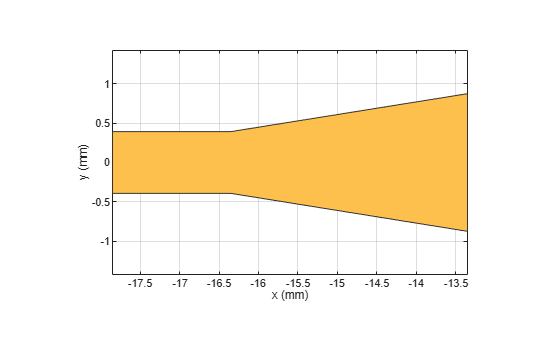#### Create SIW

Use `antenna.Rectangle` object to create the SIW. This object adds high-pass filter characteristics to the lower bound of the antenna resonance. The SIW operates at a frequency of 25 GHz.

Create series of vias to the SIW transmission line.

```siw = antenna.Rectangle(Length=l_siw, Width=w_ant, Center=[-l_ant/2+l_feed+l_taper+l_siw/2 0]); for i=1:9 viapointX(i) = viaX + (i-1)*(p_siw); viapointY(i) = -viaY; layer1(i) = 1; layer2(i) = 3; vialoc1 = [viapointX' viapointY' layer1' layer2']; end for i=1:9 viapointX(i) = viaX + (i-1)*(p_siw); viapointY(i) = viaY; layer1(i) = 1; layer2(i) = 3; vialoc2 = [viapointX' viapointY' layer1' layer2']; end ```

Create the antipodal antenna with two arms named as `topArm` and `bottomArm`. Use `antenna.Polygon` and `antenna.Rectangle` functions to create the arms of antipodal antenna with slits. Visualize the arms using the `show` function.

```topArm = antenna.Polygon; topArm.Vertices = [-l_ant/2+l_feed+l_taper+l_siw -w_ant/2;... -l_ant/2+l_feed+l_taper+l_siw -w_ant/2+w_arm;... -l_ant/2+l_feed+l_taper+l_siw+l_arm -w_ant/2]; for i = 1:20 Slit = antenna.Rectangle(Length=l_slit, Width=w_slit, Center=[slitX slitY]); slitX = slitX + 2*l_slit; topArm = topArm - Slit; end figure(Name="Top Arm") show(topArm)```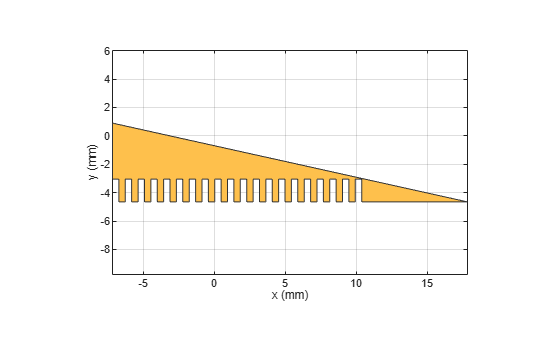Create the top layer.

`topLayer = siw + feedline + taper + topArm;`

```bottomArm = copy(topArm); bottomArm = mirrorX(bottomArm); gnd = antenna.Rectangle(Length=l_gnd, Width=w_ant, Center=[-l_ant/2+l_gnd/2 0]);```

Create the bottom layer.

```Bottomlayer = gnd + bottomArm; figure(Name="Bottom Arm") show(bottomArm)```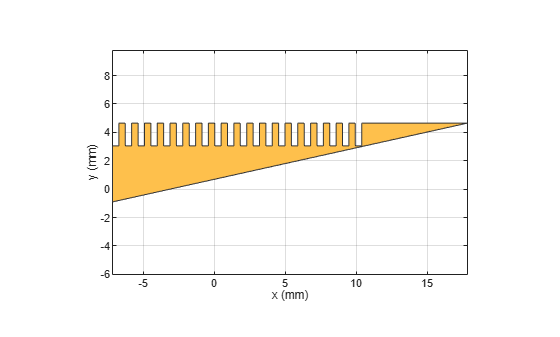#### Create Printed Circuit Board (PCB) Antenna

Use the `pcbStack` object to create a PCB antenna using the SIW filtenna geometry. You can extract the shape of the top layer by querying the `Layers` property.

Visualize the antenna using the `show` function.

```siwFiltenna = pcbStack ; substrate = dielectric("Teflon"); substrate.EpsilonR = 2.2; substrate.Thickness = 0.254e-3; siwFiltenna.BoardThickness = 0.254e-3; siwFiltenna.Layers = {topLayer,substrate,Bottomlayer}; boardShape = antenna.Rectangle(Length=l_ant, Width=w_ant, Center=[0 0]); siwFiltenna.BoardShape = boardShape; siwFiltenna.FeedDiameter = w_feed/4; siwFiltenna.FeedLocations = [-l_ant/2+w_feed/4 0 3 1]; siwFiltenna.ViaLocations = [vialoc1; vialoc2]```
```siwFiltenna = pcbStack with properties: Name: 'MyPCB' Revision: 'v1.0' BoardShape: [1x1 antenna.Rectangle] BoardThickness: 2.5400e-04 Layers: {[1x1 antenna.Polygon] [1x1 dielectric] [1x1 antenna.Polygon]} FeedLocations: [-0.0177 0 3 1] FeedDiameter: 1.9565e-04 ViaLocations: [18x4 double] ViaDiameter: [] FeedViaModel: 'strip' FeedVoltage: 1 FeedPhase: 0 Conductor: [1x1 metal] Tilt: 0 TiltAxis: [1 0 0] Load: [1x1 lumpedElement] ```
```siwFiltenna.ViaDiameter = 0.00025; siwFiltenna.FeedViaModel = "square"; siwFiltenna.FeedVoltage = 1; siwFiltenna.FeedPhase = 0; show(siwFiltenna)```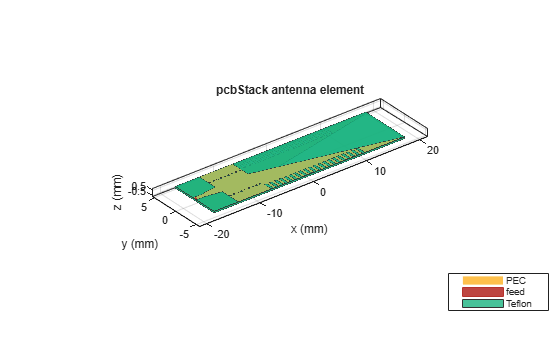### Analyze Filtenna

Use the `mesh` function to mesh the SIW filtenna. Set the maximum edge length to one eighth of the wavelength to get a fine mesh.

```plotfrequency = 40*1e9; lambda = 3e8/plotfrequency; % Wavelength mesh(siwFiltenna, MaxEdgeLength=lambda/8);```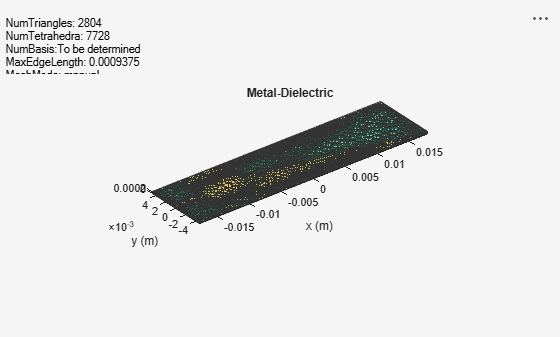#### Estimate memory requirement

Use the `memoryEstimate` function to calculate the memory required to solve the structure.

`memEst = memoryEstimate(siwFiltenna,25e9)`
```memEst = '1.5 GB' ```

#### Calculate S-Parameters

To calculate and plot the S-parameters, set the `plotSParams` variable to true. Calculating and plotting the S-Parameters can take a long time. To save time, set `plotSParams` to false and use the precomputed results in the `FilterAntennaData` MAT file attached to this example for the analysis.

```plotfrequency = 25*1e9; frequencyRange = (20:0.1:40)*1e9; plotSParams = false; if plotSParams s = sparameters(siwFiltenna,frequencyRange); rfplot(s) end```

Load the data from the `FiltennaData` MAT file, which contains the simulated and measured data in the `S11_simulated`, `S11_measured`, `pattern_simulated`, and `pattern_measured` variables. Use `S11_simulated` to plot the S-Parameters.

```load FiltennaData.mat plot(frequencyRange,S11_simulated,"r",LineWidth=2); xlabel("Frequency (Hz)"); ylabel("Reflection Coefficient");```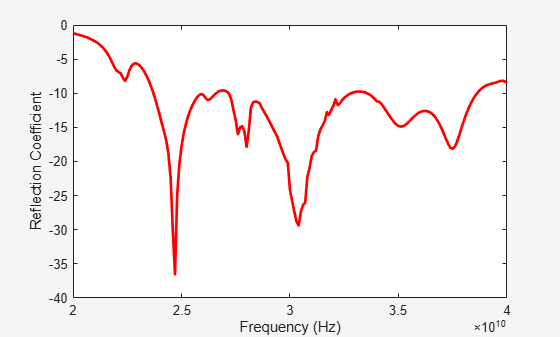Use the `pattern` function to plot the 3-D radiation pattern at 25 GHz.

`pattern(siwFiltenna,25e9);`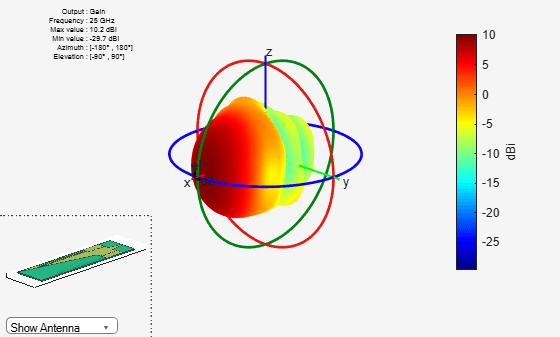Use the `pattern` function to plot the 2-D radiation pattern at an elevation angle of 0 degrees`.`

`pattern(siwFiltenna,25e9,0:1:360,0);`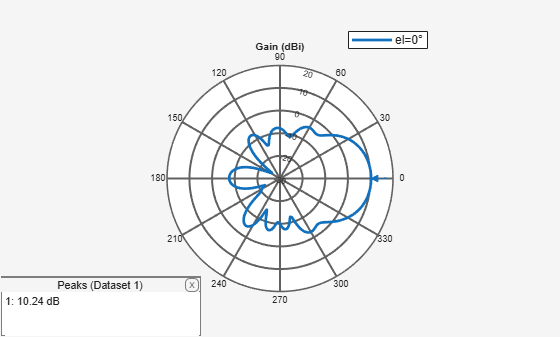Use the p`attern` function to plot the 2-D radiation pattern at an azimuth angle of 0 degrees`.`

`pattern(siwFiltenna,25e9,0,0:1:360);`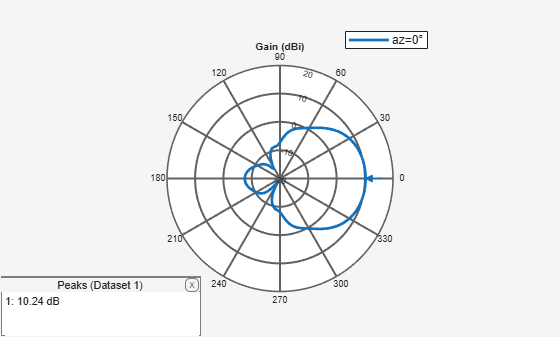#### Surface Current Distribution

Use the `current` function to plot the current distribution of the antenna at 25 GHz.

`current(siwFiltenna,25e9, scale="log");`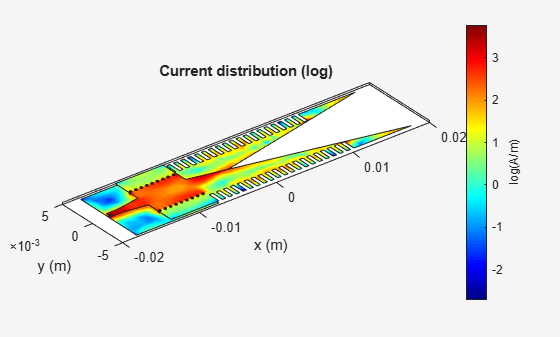### Compare Simulated and Measured Results

The SIW filtenna was built and tested for the reflection coefficient and the radiation pattern. The reflection coefficient was measured on a Keysight© N5224B PNA Network Analyzer. The radiation pattern measurements were performed in an anechoic chamber.

This figure shows the top view of the antenna.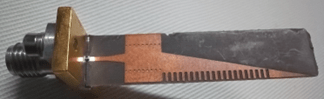This figure shows the bottom view of the antenna.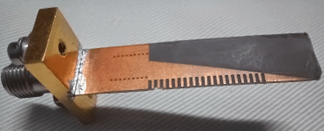Compare the simulated and measured reflection coefficients as functions of frequency.

``` plot(frequencyRange,S11_simulated,"r",LineWidth=2); hold on plot(frequencyRange,S11_measured',"b",LineWidth=2); hold off xlabel("Frequency (Hz)"); ylabel("Reflection Coefficient"); legend("Simulated","Measured");```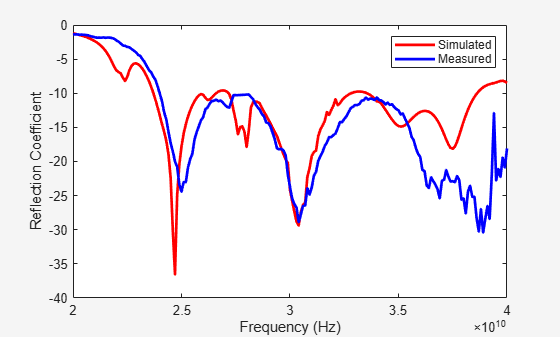Compare the simulated and measured radiation patterns as functions of azimuth angles. The proposed antenna exhibits stable radiation characteristics with a gain of 10.25 dB at 25 GHz.

``` plot(-180:1:180,pattern_simulated,"r",LineWidth=2); hold on plot(-180:1:180,pattern_measured',"b",LineWidth=2); hold off xlabel("Azimuth Angle (degree)"); ylabel("Normalized Gain"); legend("Simulated","Measured");```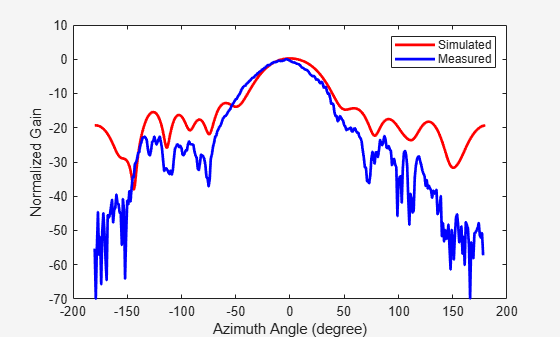### Conclusion

The designed antenna has a maximum gain of 10.25 dB and a bandwidth of 23.8 GHz to 40 GHz. You verify the efficiency of the filtenna by comparing the simulated value against a prototype that has been fabricated and measured. The measured and simulated results agree well. The filtenna is a promising candidate for compact 5G/B5G millimeter-wave communication system.

### Reference

 Mengyun Hu, Zhiqiang Yu, Jun Xu, Ji Lan, Jianyi Zhou, and Wei Hong. "Diverse SRRs Loaded Millimeter-Wave SIW Antipodal Linearly Tapered Slot Filtenna With Improved Stopband," in IEEE Transactions on Antennas and Propagation, 69, no. 12, (December 2021): 8902–7. https://doi.org/10.1109/TAP.2021.3090854.